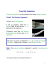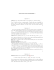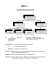Homework 2

Transcription

Homework 2
ICS 6D
Due: Wednesday, October 22, 2014, 3:00PM
Homework 2
Instructor: Sandy Irani
1. Give a recursive definition for strings of properly nested parentheses and curly braces. For example
({}){}() is properly nested but ({)} is not.
2. Let B = {0, 1}. In lecture, we discussed the recursive definition for B ∗ , the set of all binary strings
of length 0 or larger. Recall that λ is the symbol for the empty string that has no characters. Here is
the recursive definition for B ∗ :
• λ is in B ∗ .
• if x ∈ B ∗ , then x0 and x1 is also in B ∗ .
Give a recursive definition for the set S which is all binary strings in which all the 0’s come before all
the 1’s. For example 0011111 ∈ S, but 0010111 6∈ S.
3. Suppose someone takes out a home improvement loan for \$30,000. The annual interest on the loan
is 6% and is compounded monthly. The monthly payment is \$600. Let an denote the amount owed
at the end of the nth month. The payments start in the first month and are due the last day of every
month.
(a) Give a recurrence relation for an . Don’t forget the base case.
(b) Suppose that the borrower would like a lower monthly payment. How large does the monthly
payment need to be to ensure that the amount owed decreases every month?
4. Give a recursive algorithm that takes as input two positive integers x and y and returns the product
of x and y. The only arithmetic operations your algorithm can perform are addition or subtraction.
Furthermore, your algorithm should have no loops.
5. Give a recursive algorithm that takes as input a non-negative integer n and returns a set containing all
binary strings of length n. Here are the operations on strings and sets you can use:
• Initialize an empty set S.
• Add a string x to S.
• y := 0x (This operation adds a 0 to the beginning of string x and assigns the result to string y).
• y := 1x (This operation adds a 1 to the beginning of string x and assigns the result to string y).
• return(S)
Also, you can have a looping structure that performs an operation on every string in a set:
For every x in S
//perform some steps with string x
6. Give a recursive algorithm whose input is a, a real number and n a positive integer and whose output
n
is a2 . Note that the exponent of a is 2n .
For each of the inductive proofs below, clearly indicate the base case and inductive step. For the inductive
step, state what you are assuming and what you are proving and show where you use the inductive hypothesis
2
1. Prove that for any n ≥ 1, 4 evenly divides 32n − 1.
2. Prove that for any n ≥ 1, 6 evenly divides 7n − 1.
3. Prove that for every positive integer n,
Pn
4. Prove that for every positive integer n,
Pn
5. Prove that for n ≥ 2, n3 ≥ 2n + 4.
6. Prove that for n ≥ 2, 3n > 2n + n2 .
7. Define the sequence {cn } as follows:
• c0 = 5
• ck = (ck−1 )2 .
n
Prove that for n ≥ 0, cn = 52 .
8. Define the sequence {bn } as follows:
• b0 = 1
• bk = 2 · bk−1 + 1.
Prove that for n ≥ 0, bn = 2n+1 − 1.
3
j=1 j =
j=1 j
n(n+1)
2
2
.
· 2j = (n − 1) · 2n+1 + 2.

CIS 265 Homework 3 Due: Th. Mar 19th Fall 2015 â Prof. Matos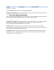Basic Information - Tecnológico de Monterrey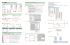Assignment 2 - Technische Universiteit Eindhoven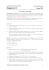Test 1 Solutions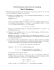Sample Problems = +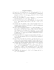CMSC 28100-1 Spring 2015 Homework 2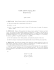Flemish String Board Attachment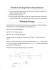Sample Problems Math 334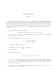Sample Final Exam Questions (Mandatory Part)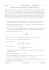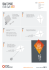Installation instructions Finetune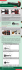Homework #3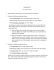HOW TO CHANGE STRINGS ON A VB WITH THE HIPSHOT SYSTEM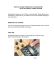AwesomeMath Admission Test Cover Sheet Your Name Admission Test Check oneZoë and her Zebra Mobile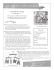MATH5011 Real Analysis I Exercise 8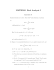2015 Exam - MAA Sections - Mathematical Association of America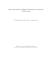CS 241 Homework 5 Xiguo Ma Foundations I Due: Week 11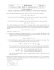Homework 2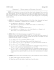Proof By Induction